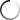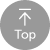B1 中級 4

 Let's talk about accessing the values in an array using the four EQ functions the four each function essentially steps through an array, and for all the values in the array, it can turn those values into variables. And then you can test against those variables, so you can use this for things like If l statements, you do four each. You give the array name, you turn the values and variables, and then depending on if those variables equal or do not equal something, an event could be triggered. Or you can simply use for each to do something, such as list out all the names. So the example that I'm going to show you is I have a list of names, possibly people coming to an event membership list. Something like that, and what it will do is four. Each will go through, and for each one of the values, it will turn the value into a variable. And then we will print the variable out. So it's kind of like a loop on Lee when you're dealing with a raise, and this allows you to access the values in the array, turn those values and the variables so that you could do something with them within Ph. P. So with that, let's go over the computer so I can give you a demonstration to show you how four each works for getting values out of a raise. So I've created this basic four each dot PHP script. And with this we have a simple array here. I have not named the Keys. I have just added basically, people's names Think of this is like a list of people coming to an event, and I've just people put those people in there. So what we've done again, we open up with PHP tag and we're going to create the variable. So dollar sign names and we're going to set the value of dollar sign names to the array. And then we're going to put in these different people's names. So again you put single quotation mark the name close single quotation mark comma and you continue all the way through. So we put in Bob. We put in 10. We put in Tommy putting Shelly. He put it, Julia, we closed the parentheses. And then, of course, we do the semi colon. So this has created the array \$4 sign names and set the value of position zero to bob. Position one to Tim. Position to Tom. Position three to Shelly and position for two. Julia. Now all we're going to Dio very simply for this is we're going to use the four each function. We're going to four each, Then we're going to open parentheses, and the first thing we do with the four each function is we feed it the array that we're going to use. So for this theory that we're using is names, and so we're going to feed it names, and then he simply do put as so names as and then whatever variable you put here and notice I haven't created this variable forehand beforehand. You can create this variable right here. And so what this is going to do is whatever variable you put here, it's going to take the value from the array. And it's going to make that value the value of this variable. So basically four eat the first time this goes through, it will take Bob and so dollar sign value. It will be set to the value of Bob. Then it will be sent to the value of Tim will be set to the value of Tom, Shelly and Julia. So we feed it the array name we say as then we say what we want. The variable will be called for the value that's being fed two for each. And then we go from there past that. We just simply close the parentheses. And then we open the squiggly brackets of squiggly bracket. Basically, everything between the two squiggly brackets will will run for every value in the array. So I could have 100 lines of code here if I want it. All we're going to be doing right now is just doing a print. But understand, you could put if l statements. But here you could but put a whole bunch of different code for every value in the array. So we're going to Dio is we're going to simply say, print double quotation marks, dollar sign value, and then we're going to do a break. So basically what this will do is for every value in the array, it will print that value, and then it will break to the next nine to the next night. The next line, Basically it's a very simple thing here. Then we close the double quotation marks. Of course, we do the semi colon, and then we close the squiggly brackets now to do a little bit a little bit more with this. What I've done is I printed an additional brake line, so basically this array will print out, and then there will be a gap print in additional brake line. And now what I'm going to do is I'm going to sort the array. So again, if this is a report for people coming to an event, so I'm going to use the sort function I'm going to give sort the name's array, and then I'm going to use the semi colon. And now, now that we've sorted so sore sorts, the array in alphabetical order, now we're going to do for each the exact same thing names as value, squiggly bracket. Then we're going to print out the value of dollar sign value, close squiggly bracket, and then we're going to close the PHP. So for this, what's going to happen is we haven't done anything to the array yet, so this is going to print out the array as it looks as we coated it in then was going to happen here is we're going to sort the array alphabetically, and then we're going to print it out after we've sorted that. So now if we go to a Web browser, we put in Silicon dough Joe, then we put at four each. Now what we can see is we can see this array gets printed out. So we see Bob, Tim, Tom, Shelly, Julia. So this gets printed out, each value it's printed out on the line, and then we have a break, and then we see that it gets sorted alphabetically. So now it's Bob, Julia, Shelly, Tim and Tom. So this is a basic way that you can access the values and an array. If you don't know the position of the values again so you could have an if so, you could have this go through. And then if the name equals Bob, then do something that's type of stuff that you can d'oh once you're able to turn those values in an array into actual variables. So that's all there is to using four EQ in order to access the values of an array in PHP And so with this, what you're able to do is you're able to turn those values into variables, and then you're able to test against those variables or print out the variables so on and so forth. So this is incredibly, ah, easy way that you could use a raise again in order to do simple projects such as attendance lists or anything like that. If you're gonna be doing inventory control, if you're going to be doing a lot of different types of things. This is a way you can print out from the array, and you can actually test against the values in a ready and do certain things. So that's all there really is to using four EQ in order to access the values in an array, and that's why it's important.

# PHP - Access Array Values - foreach()

4

#### 影片學習單字重點#### 影片討論1. ## 1. 單字查詢

在字幕上選取單字即可即時查詢單字喔！

2. ## 2. 單句重複播放

可重複聽取一句單句，加強聽力！

3. ## 3. 使用快速鍵

使用影片快速鍵，讓學習更有效率！

4. ## 4. 關閉語言字幕

進階版練習可關閉字幕純聽英文哦！

5. ## 5. 內嵌播放器

可以將英文字幕學習播放器內嵌到部落格等地方喔

6. ## 6. 展開播放器

可隱藏右方全文及字典欄位，觀看影片更舒適！

1. ## 英文聽力測驗

挑戰字幕英文聽力測驗！

1. 點擊展開筆記本讓你看的更舒服

1. UrbanDictionary 俚語字典整合查詢。一般字典查詢不到你滿意的解譯，不妨使用「俚語字典」，或許會讓你有滿意的答案喔